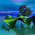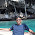Jul 15, 2013

Math Game of Zero String

Source: Quantnet

Problem:

You have a string of bits. You scan from right to left.

If you encounter a '1', you have the option to flip it to a 0 or keep it as is.

If you encounter a '0', your adversary has the option to flip it to a 1 or keep it as is.

Your goal is to zero all the bits once you reach the end of a scan (i.e. at the left most bit), whilst you adversary wishes to prolong the game indefinitely.

We continually re-scan until we reach the aforementioned goal state.

Can you prove that the game will eventually terminate?

1.Base Case: You trivially win if there are 0 bits. You easily win if there's 1 bit.

Step:
1. Assume you can win when there are N-1 bits, for some N>1.
2. To win on a sequence of size N, simulate winning on the first N-1 bits.
3. Once the first N-1 bits are all 1, the last bit is either 0 or 1.
4. If the last bit is 0, flip it to 1 to win.
5. If the last bit is 1 and the adversary does not flip it you win.
6. If the last bit is 1 and the adversary flips it, go to step 2.

Step 6 can only be reached once, because when step 6 is reached you gain control of the last bit and will win when step 4 is reached for the second time.

Therefore, by induction, you can force all bits to be 1 for any finite constant sized sequence of bits.

1.Here you are assuming that in order to win, all the digits should be 1 just before.
This, according to me is an incorrect assumption.
Eg. tell me how will you play for 10101101.

2.Rough Sketch of proof:

We describe a procedure that leads to our win for any arbitrary binary string of length k.

Assume you have some X 0's and Y 1's to begin with, where X and Y are natural numbers.

Procedure:

On each of your moves, we break into two cases depending on all your opponent's moves leading up to that move:

a) If your opponent hasn't changed any "0"s to "1"s before this point, then change this particular "1" to a "0"

b) If your opponent has changed a "0" to a "1", then leave this particular "1" unchanged

Repeat until you win.

Why this procedure works: Let's call the rightmost "1" in the starting bit sequence "special" (excluding the leftmost bit).

Now note your opponent is guaranteed to lose after making move i (position i)in the number if all bits to the left of i are one, and all bits before i and including i are zero. Thus by following the above procedure, either we get an additional "1" to the right of the "special", OR our opponent changes a "0" that is left of the "special" and all the other "1"s that came before it become zero.

Now note that this second case must eventually come true, we always must get a new "special" that is to the left of the original "special" (because there are a finite number of digits that come before the "special" - just think about it). When this happens, the new "1" that is formed is the new "special".

Thus all "1"s must migrate to the left after some number of repetitions. Once the leftmost bit in the string becomes a "1", it will never be changed until the winning repetition (because the only case in which it would be changed is if every bit before it was zero). Thus once the leftmost bit is a "1", we can ignore it and pretend we have a string of length one bit shorter. If we keep repeating, all the bits must eventually become one. When this happens we can zero out every bit in one move.

1.excellent!!

2.3.Base case : trivial (for 0 and 1 bit)
Let us assume there exists a strategy to win n-1 bits game (i.e for any possible combinatorial n-1 bits sequence can be converted to a sequence that contains (n-1) 0s) . For clarity let me call this winning state as W.

For n bit game , we will use same strategy to reach a W where I have won the right most n-1 bits , leaving the left most nth bit . I can reach this state W because we are scanning from right to left or in layman terms i will play as if i didn't knw the most significant bit (MSB) exists or not.

Now if the MSB is 1 (implies i have the control , so i can flip and win ) : trivial
if MSB is 0 : and given that the adversary tries to prolong indefinitely : the only option for adversary is to flip this to 1 (else I win by default because i am on the last bit , which is still 0 and my previous n-1 bits are already 0). Once the MSB is flipped to 1 (I again get the total control on MSB , so keep it aside or ignore it as if it doesn't exist anymore ) . Now we are left with n-1 bits all 0s and MSB which we do not care about as 1. Now whatever the adversary may do with the n-1 0 bits in the next turn , I can come back to the W (winning) state where all n-1 bits are 0. Once I reach W state , and because MSB is 1 , i can just flip it to win.

The solution is based on induction but the key insight is the problem is reduced to a smaller size problem as soon as the MSB is flipped to 1.. Once MSB becomes 1 , the adversary can do nothing about it and the game can be played as if its a n-1 bit game , but to force the adversary to flip MSB to 1 (if it was originally 0) , you have to win the n-1 bit game.

4.base case : trivial
let us say we know how to win n-1 bit game - Assumption 1
for n bit game , we will use the same strategy to win the n-1 bits and ignore the most significant bit.
once we win the n-1 bit :

if MSB is 1 ( we have control over this bit) , so we flip and win
if MSB is 0 ( given adversary wants to prolong indefinitely) , the only option for adversary is to flip it , else we will win , since all n bits will be 0. If the adversary flips to 1 (good for us , because it gives us the control over the MSB , so we keep it aside or maybe even hide it , doesn't really matter because adversary can do nothing about this bit anymore since it is 1 until unless flipped to 0 lol) . Now we are back to n-1 bit game (the only thing worth noting is that we are starting from a winning state (because n-1 bits are already 0). Whatever the move adversary takes , we can get back to the winning state from our initial assumption 1. So once we are back to the winning state with n-1 bits , we can flip the MSB (since it was 1 all this while ) to win the n bit game.

5.Not sure how to prove it mathematically, but if you move from right-to-left, and you encounter a 1, and there are no 1 bits to the right of it, flip it to 0. No matter what the opponent tries to do, this strategy will win every time.

Fraction Brainteaser

Source: Sent to me by Gaurav Sinha Problem: Siddhant writes a Maths test and correctly answers 5 out of 6 Arithmetic questions and 20...